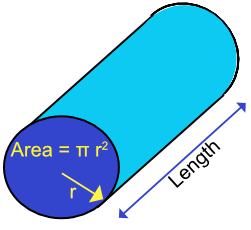Volume of a Cylinder

# Volume of a Cylinder

GCSE(F), GCSE(H),

A cylinder is a circular prism. As with other prisms, the volume of a cylinder can be calculated using the cross-sectional area x length.

Th cross-section of a cylinder is a circle. As the area of a circle =πr^2, the volume of a cylinder is πr^2l, where r is the radius of the circular end, and l is the length of the cylinder.## Examples

1. What is the volume of a cylinder with a diameter of 4cm and a length of 42cm? Give your answer correct to 1 decimal place.

The radius of a circle is frac(1)(2) the diameter = frac(1)(2) xx 4 = 2cm

The cross-sectional area is pir^2 = pi xx 2^2 = 4pi

The volume of the cylinder = cross-sectional area x volume

= 4pi xx 42

= 527.79 cm3

2. What is the diameter of a cylinder with a volume of 1000cm3 and a length of 100cm? Give the answer correct to 1 decimal place.

Volume of a cylinder = pir^2l
1000 = pir^2 xx 100
10 = pir^2
r^2 = 3.183